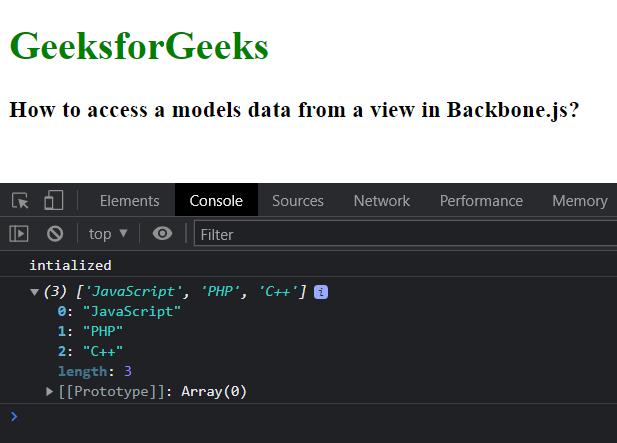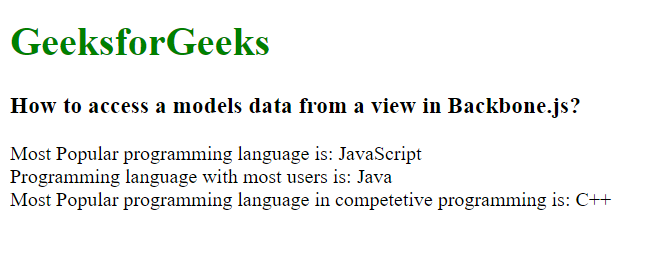Open in App
Not now

# How to access a models data from a view in Backbone.js ?

• Last Updated : 25 Oct, 2022

Backbone.js is a compact library used to organize JavaScript code. An MVC/MV* framework is another term for it. If MVC is unfamiliar to you, it simply refers to a technique for designing user interfaces. The creation of a program’s user interface is made considerably easier by JavaScript functions. BackboneJS provides a variety of building elements to aid developers in creating client-side web applications, including models, views, events, routers, and collections.

A model is the main part of any JavaScript Application, holding both the interactive data and the majority of the logic necessary to support it, including conversions, validations, computed properties, and access control. To access the model’s data from a view, you need to initialize the model and create an object of the model in the view.

Syntax:

```var demoModel= Backbone.Model.extend({
initialize: function(){
...
},
...
})
var demoView = Backbone.View.extend({
initialize: function() {
this.model = new demoMode;();
},
...
});
var demo = new demoView();```

Example 1: The code below demonstrates how we can apply the above-given approach and print the data in the JavaScript console.

## HTML

 `` `<``html``>`   `<``head``>` `    ``<``title``>How to access a models data ` `          ``from a view in Backbone.js?` `    ``<``script` `src``=` `"https://code.jquery.com/jquery-2.1.3.min.js"` `type``=``"text/javascript"``>` `    ``` `    ``<``script` `src``=` `"https://cdnjs.cloudflare.com/ajax/libs/underscore.js/1.8.2/underscore-min.js"` `            ``type``=``"text/javascript"``>` `    ``` `    ``<``script` `src``=` `"https://cdnjs.cloudflare.com/ajax/libs/backbone.js/1.1.2/backbone-min.js"` `            ``type``=``"text/javascript"``>` `    ``` ``   `<``body``>` `    ``<``h1` `style``=``"color: green;"``>` `        ``GeeksforGeeks` `    ```   `    ``<``h3``>How to access a models data from` `          ``a view in Backbone.js?`   `    ``<``script` `type``=``"text/javascript"``>` `        ``var lang = Backbone.Model.extend({` `            ``initialize: function(){` `                ``console.log('initialized');` `            ``},` `            ``defaults:{` `                ``names:['JavaScript','PHP','C++']` `            ``}` `        ``})` `        ``var lang_view = Backbone.View.extend({` `            ``initialize: function() {` `                ``this.model = new lang();` `                ``this.outFunc();` `            ``},` `            ``outFunc: function(){` `                ``console.log(this.model.get('names'))` `            ``}` `        ``});`   `        ``var myView = new lang_view();` `    ``` ``   ``

Output:Example 2: The code below demonstrates how we can access the model’s data and use it in the view.

## HTML

 `` `<``html``>`   `<``head``>` `    ``<``title``>` `          ``How to access a models data from ` `          ``a view in Backbone.js?` `      ``` `    ``<``script` `src``=` `"https://code.jquery.com/jquery-2.1.3.min.js"` `type``=``"text/javascript"``>` `    ``` `    ``<``script` `src``=` `"https://cdnjs.cloudflare.com/ajax/libs/underscore.js/1.8.2/underscore-min.js"` `            ``type``=``"text/javascript"``>` `    ``` `    ``<``script` `src``=` `"https://cdnjs.cloudflare.com/ajax/libs/backbone.js/1.1.2/backbone-min.js"` `            ``type``=``"text/javascript"``>` `    ``` ``   `<``body``>` `    ``<``h1` `style``=``"color: green;"``>` `        ``GeeksforGeeks` `    ```   `    ``<``h3``>` `          ``How to access a models data ` `          ``from a view in Backbone.js?` `      ```   `    ``<``script` `type``=``"text/javascript"``>` `        ``var lang = Backbone.Model.extend({` `            ``initialize: function(){` `                ``console.log('initialized');` `            ``},` `            ``defaults:{` `                ``names:['JavaScript','Java','C++']` `            ``}` `        ``})` `        ``var lang_view = Backbone.View.extend({` `            ``initialize: function() {` `                ``this.model = new lang();` `                ``this.outFunc();` `            ``},` `            ``outFunc: function(){` `                ``var values = new Array();` `                ``values = this.model.get('names');` `                ``console.log(` `                      ``"Most Popular programming language is: " ` `                    ``+ values + "<``br``>"),` `                ``console.log(` `                      ``"Programming language with most users is: " ` `                    ``+ values + "<``br``>"),` `                ``console.log(` `                      ``"Most Popular programming language in "` `                      ``+ "competetive programming is: "` `                    ``+ values + "<``br``>")` `            ``}` `        ``});`   `        ``var myView = new lang_view({});` `    ``` `` ``

Output:Reference: https://backbonejs.org/#Model

My Personal Notes arrow_drop_up
Related Articles